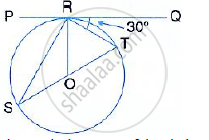Share

# If Pq is a Tangent to the Circle at R; Calculate: - Mathematics

Course

#### Question

If PQ is a tangent to the circle at R; calculate:
(i) ∠PRS

(ii) ∠ROTGiven :  O is the centre of the circle and angle TRQ = 30°

#### Solution

PQ is a tangent and OR is the radius.

∴ OR ⊥  PQ

∴ ∠ ORT = 90°

⇒∠ TRQ = 90° - 30° = 60°

But in Δ OTR ,

OT = OR (Radii of the same circle)

∴ ∠ OTR = 60° Or ∠ STR = 60°

But,

∠ PRS = ∠ STR = 60 (Angle in the alternate segment)

In ΔORT,

∠ ORT  = 60°

∠ OTR =60°

∴ ∠ ROT = 180° - (60° +60° )

∴ ∠ ROT = 180°  - 120° = 60°

Is there an error in this question or solution?